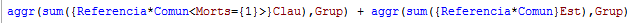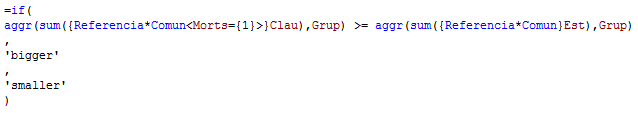# QlikView App Dev

Discussion Board for collaboration related to QlikView App Development.

Announcements
Skip the ticket, Chat with Qlik Support instead for instant assistance.
cancel
Showing results for
Did you mean:Not applicable

## AGGR Problem Comparison

Hi,

As I know, Qlikview permits to operate with 2 aggr expresions, for example:So, QV will sum the 1st expresion + the 2nd expresion for each "Grup" dimension. That's perfect!

But, the problem appears when we want to compare both aggr, for example:Here, QV only compares the first group of the dimension called "Grup" and applies the result for all the other "Grup" values. So, QV does not do the comparison for  every single "Grup" value.

Do you know any other way to compare 2 aggr?

1 Solution

Accepted SolutionsSpecialist

sum(

Aggr(

if(sum({Referencia*Comun<Morts={1}>}Clau>=Sum({Referencia*Comun}Est)

,
(sum({Activitat*Comun<Morts={1}>}Clau) * Sum({Referencia*Comun<Morts={1}>}Clau) /                Sum({Referencia*Comun}Clau))

,

Sum({Activitat*Comun<Morts){1}>}Clau)

)

, Grup)

)

9 RepliesMVP

Hi,

like

sum(Aggr(Sum()))

=if(

sum(Aggr(Sum({Referencia*comun<Morts={1}>}Clau),Grup)) >= sum(Aggr(Sum({Referencia*comun}Est),Grup))

,

'Bigger'

,

'Smaller'

)

Regards

Great dreamer's dreams never fulfilled, they are always transcended.Specialist

Where are you doing this comparison?

if Grup is the dimensjon in your chart then a simple

if(Sum(Clau)>=Sum(Est),'Bigger','Smaller') should work fine.

if you have other dimensions in your chart you will have to use the NODISTINCT modifier to get the result of the aggr over more than one record per Grup.

Aggr(Nodistinct if(Sum(Clau)>=Sum(Est), 'Bigger', 'Smaller'), Grup)Not applicable
Author

Hi,

Thank for response.

If a put "sum" before the "aggr" QV will aggregate each expresion by the dimension "Grup" and I QV will compare only 2 numbers, 1 for each expresion. And what I want is that QV compares all the possible values of the dimension "Grup" for both expresions, without aggregating the result.Not applicable
Author

Exacly, the problem is that I need this comparison in a text object, but not in a chart.

thank you for response.Specialist

Well, you are trying to compare an Array to another Array and display only one value.

What is the expected output?

=if(sum(Aggr(sum(Clau),Grup)) >= sum(Aggr(sum(Est),Grup)), 'Bigger', 'Smaller')Not applicable
Author

The real formula is:The expected output is a single value. I need the "If comparison" because there 2 ways of calculations, depending of the result of the initial condition.Partner - Champion III

Hi

you can only add two Aggr() expressions if they both return a single value, so you adding two scalar values, not two lists. If they return a list of value, rather than just a single value, the addition returns NULL.

So adding two scalar values does not require an Aggr() expression. If the table has Grup as a dimension, the table dimension will take care if the grouping, and you can just compare the two sums inside the Aggr() expressions with each other.

HTH

Jonathan

Logic will get you from a to b. Imagination will take you everywhere. - A EinsteinSpecialist

sum(

Aggr(

if(sum({Referencia*Comun<Morts={1}>}Clau>=Sum({Referencia*Comun}Est)

,
(sum({Activitat*Comun<Morts={1}>}Clau) * Sum({Referencia*Comun<Morts={1}>}Clau) /                Sum({Referencia*Comun}Clau))

,

Sum({Activitat*Comun<Morts){1}>}Clau)

)

, Grup)

)Not applicable
Author

Woooow yes!!!...I have checked and that's exactly what I need. Thank you SimenCommunity Browser